Succeed with maths – Part 1

Start this free course now. Just create an account and sign in. Enrol and complete the course for a free statement of participation or digital badge if available.

Free course

# 3 Addition and subtraction on the number line

The number line can be used to show how calculations can be carried out with negative numbers. First you’ll have a reminder of how this works with positive numbers by looking at the sum 2 + 4.

Using Figure 7 below you can see that to add 4 onto 2 you need to move 4 places to the right of 2, giving 6.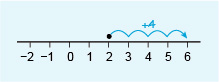Figure 7 The sum 2 + 4 = 6

The same method of moving to the right works if the starting number is negative. For example, suppose an account is overdrawn by £5, the account balance would be recorded as –£5. If the account holder then paid £8 into the account, £5 of this would pay off the debt and £3 would be recorded as the new balance, so (–5) + 8 = 3.

On the number line in Figure 8, the starting number is –5, and moving 8 units to the right verifies the answer as 3.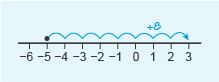Figure 8 The sum (–5) + 8 = 3

Now consider subtraction, again first with positive numbers, for example 6 − 4. The starting number is 6 but this time you move 4 units to the left to get to the answer, which is 2 (Figure 9). To subtract, you move to the left on the number line.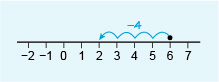Figure 9 The sum 6 − 4 = 2

Similarly, if an account is overdrawn by £2 (–£2), and then the account holder withdrew another £3, the account would be overdrawn by £5. This is represented on the number line by starting at –2, then moving 3 units to the left to get the answer –5. This is written as (–2) − 3 = –5 (Figure 10).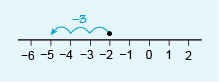Figure 10 The sum (–2) − 3 = –5

See how you get on in this next activity.

## Activity 4 Working with negative numbers

Timing: Allow approximately 10 minutes

Try the following examples. Start by drawing your own number line from –10 to + 10.

• a.3 − 7

• a.Start at 3, move 7 units to the left.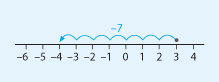Figure 11 Number line for Activity 4, part (a)

Thus, 3 − 7 = –4.

• b.(–2) + 5

• b.Start at –2, move 5 units to the right.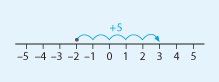Figure 12 Number line for Activity 4, part (b)

Thus, (–2) + 5 = 3.

• c.(–3) − 6

• c.Start at –3, move 6 units to the left.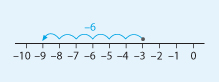Figure 13 Number line for Activity 4, part (c)

Thus, (–3) − 6 = –9.

• d.(–4) + 2

• d.Start at –4, move 2 units to the right.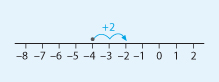Figure 14 Number line for Activity 4, part (d)

Thus, (–4) + 2 = –2.

Well done for completing your first calculations using negative numbers. These examples all involved subtracting or adding positive numbers to a negative number. Things become slightly more challenging when looking at adding and subtracting negative numbers but again you’re going to call into help your trusty number line.